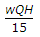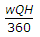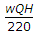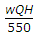# Civil Engineering - Water Supply Engineering

### Exercise :: Water Supply Engineering - Section 4

36.

The permissible amount of nitrites present in potable water, is

 A. 10 ppm B. 15 ppm C. 20 ppm D. 45 ppm E. Nil.

Explanation:

No answer description available for this question. Let us discuss.

37.

Water having pH value as 6, is

 A. alkaline B. acidic C. neutral D. none of these.

Explanation:

No answer description available for this question. Let us discuss.

38.

Alum is chemically

 A. Copper sulphate B. Aluminium sulphate C. Ferrous sulphate D. Ferric sulphate.

Explanation:

No answer description available for this question. Let us discuss.

39.

If w is the weight of water per cubic metre, Q is the discharge in cubic metres per sec and H is the total head, the required water horse power of the pump, is

 A.B.C.D.E. none of these.

Explanation:

No answer description available for this question. Let us discuss.

40.

Perched acquifers are generally found

 A. on the surface of the ground B. below the surface of the ground but above water table C. below the water table D. all the above.